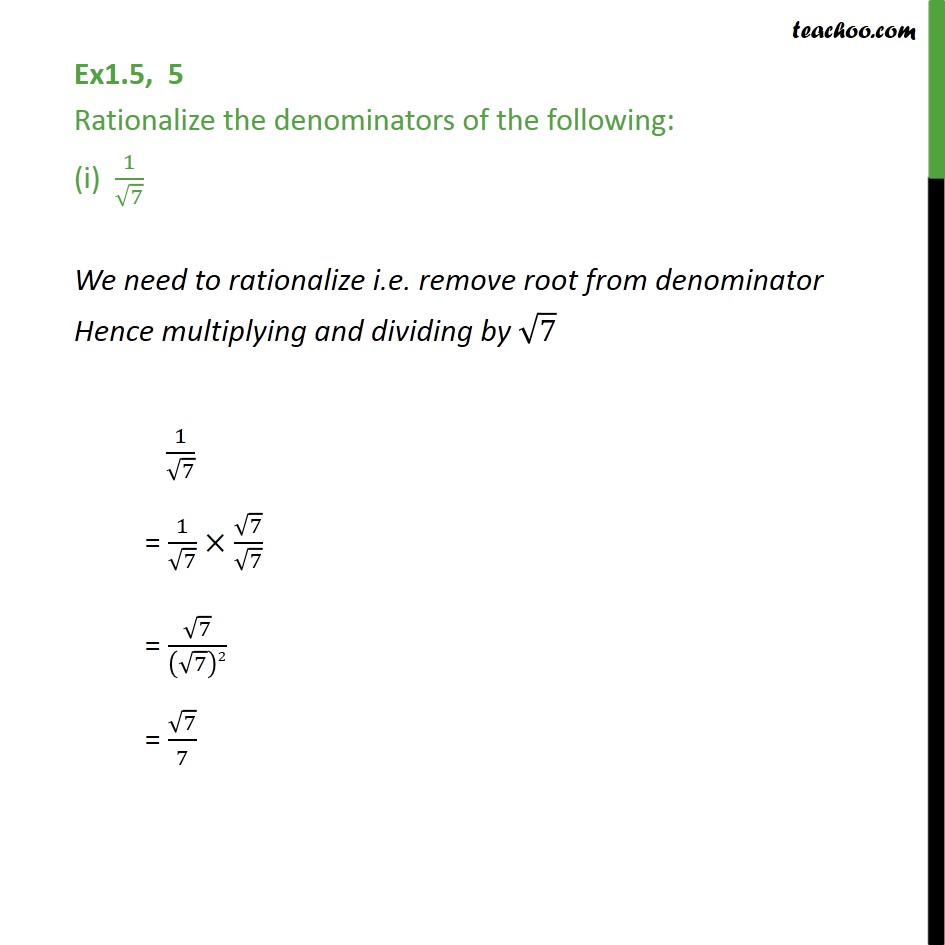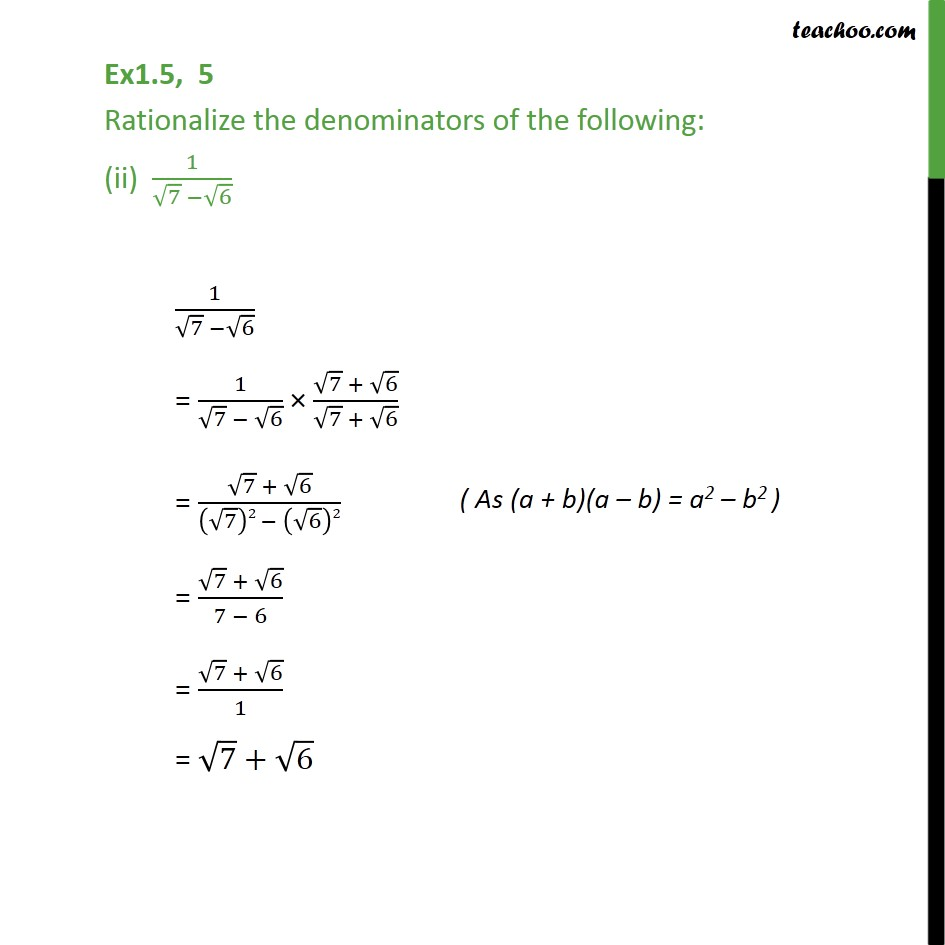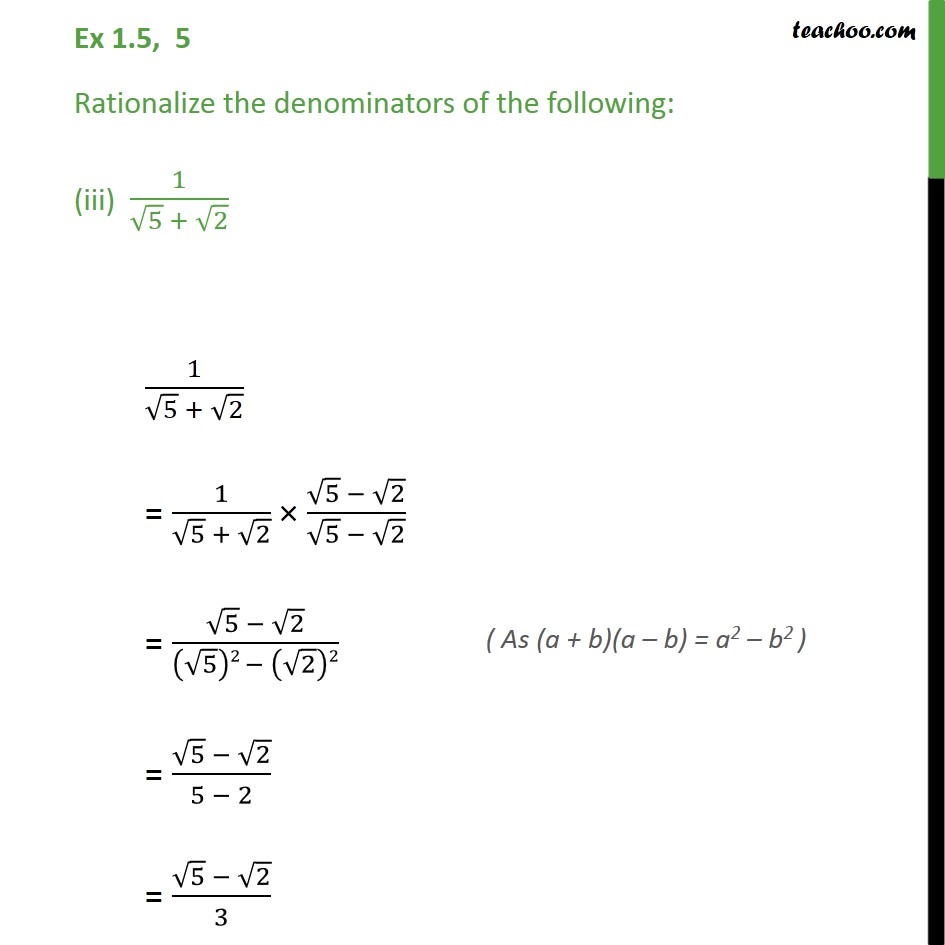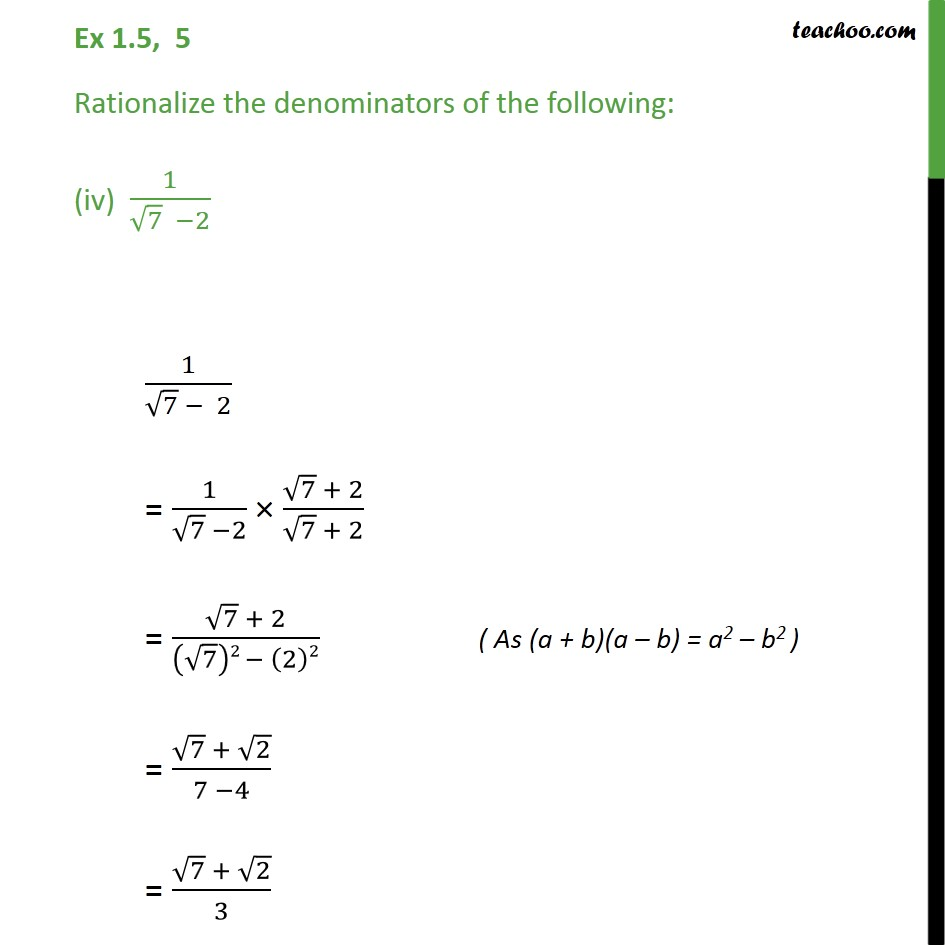Learn All Concepts of Chapter 1 Class 9 - FREE. Check - Chapter 1 Class 9 Maths1. Chapter 1 Class 9 Number Systems
2. Serial order wise
3. Ex 1.5

Transcript

Ex1.5, 5 Rationalize the denominators of the following: (i) 1/√7 We need to rationalize i.e. remove root from denominator Hence multiplying and dividing by √7 1/√7 = 1/√7 ×√7/√7 = √7/(√7)2 = √7/7 Ex1.5, 5 Rationalize the denominators of the following: (ii) 1/(√7 −√6) 1/(√7 −√6) = 1/(√7 − √6) × (√7 + √6)/(√7 + √6) = (√7 + √6)/((√7)2 − (√6)2) = (√7 + √6)/(7 − 6) = (√7 + √6)/1 = √7+√6 Ex 1.5, 5 Rationalize the denominators of the following: (iii) 1/(√5 + √2) 1/(√5 + √2) = 1/(√5 + √2) × (√5 − √2)/(√5 − √2) = (√5 − √2)/((√5)2 − (√2)2) = (√5 − √2)/(5 − 2) = (√5 − √2)/3 Ex 1.5, 5 Rationalize the denominators of the following: (iv) 1/(√7 −2) 1/(√7 − 2) = 1/(√7 −2) × (√7 + 2)/(√7 + 2) = (√7 + 2)/((√7)2 − (2)2) ( As (a + b)(a – b) = a2 – b2 ) = (√7 + √2)/(7 −4) = (√7 + √2)/3

Ex 1.5